# gptools.kernel package¶

## gptools.kernel.core module¶

Core kernel classes: contains the base Kernel class and helper subclasses.

class gptools.kernel.core.Kernel(num_dim=1, num_params=0, initial_params=None, fixed_params=None, param_bounds=None, param_names=None, enforce_bounds=False, hyperprior=None)[source]

Bases: object

Covariance kernel base class. Not meant to be explicitly instantiated!

Initialize the kernel with the given number of input dimensions.

When implementing an isotropic covariance kernel, the covariance length scales should be the last num_dim elements in params.

Parameters: num_dim : positive int Number of dimensions of the input data. Must be consistent with the X and Xstar values passed to the GaussianProcess you wish to use the covariance kernel with. Default is 1. num_params : Non-negative int Number of parameters in the model. initial_params : Array or other Array-like, (num_params,), optional Initial values to set for the hyperparameters. Default is None, in which case 1 is used for the initial values. fixed_params : Array or other Array-like of bool, (num_params,), optional Sets which hyperparameters are considered fixed when optimizing the log likelihood. A True entry corresponds to that element being fixed (where the element ordering is as defined in the class). Default value is None (no hyperparameters are fixed). param_bounds : list of 2-tuples (num_params,), optional List of bounds for each of the hyperparameters. Each 2-tuple is of the form (lower, upper). If there is no bound in a given direction, it works best to set it to something big like 1e16. Default is (0.0, 1e16) for each hyperparameter. Note that this is overridden by the hyperprior keyword, if present. param_names : list of str (num_params,), optional List of labels for the hyperparameters. Default is all empty strings. enforce_bounds : bool, optional If True, an attempt to set a hyperparameter outside of its bounds will result in the hyperparameter being set right at its bound. If False, bounds are not enforced inside the kernel. Default is False (do not enforce bounds). hyperprior : JointPrior instance or list, optional Joint prior distribution for all hyperparameters. Can either be given as a JointPrior instance or a list of num_params callables or py:class:rv_frozen instances from scipy.stats, in which case a IndependentJointPrior is constructed with these as the independent priors on each hyperparameter. Default is a uniform PDF on all hyperparameters. ValueError If num_dim is not a positive integer or the lengths of the input vectors are inconsistent. GPArgumentError if fixed_params is passed but initial_params is not.

Attributes

 num_free_params Returns the number of free parameters. free_param_idxs Returns the indices of the free parameters in the main arrays of parameters, etc. free_params Returns the values of the free hyperparameters. free_param_bounds Returns the bounds of the free hyperparameters. free_param_names Returns the names of the free hyperparameters.
 num_params (int) Number of parameters. num_dim (int) Number of dimensions. param_names (list of str, (num_params,)) List of the labels for the hyperparameters. enforce_bounds (bool) If True, do not allow hyperparameters to be set outside of their bounds. params (Array of float, (num_params,)) Array of parameters. fixed_params (Array of bool, (num_params,)) Array of booleans indicated which parameters in params are fixed. hyperprior (JointPrior instance) Joint prior distribution for the hyperparameters. param_bounds (CombinedBounds) The bounds on the hyperparameters. Actually a getter method with a property decorator.
param_bounds
__call__(Xi, Xj, ni, nj, hyper_deriv=None, symmetric=False)[source]

Evaluate the covariance between points Xi and Xj with derivative order ni, nj.

Note that this method only returns the covariance – the hyperpriors and potentials stored in this kernel must be applied separately.

Parameters: Xi : Matrix or other Array-like, (M, D) M inputs with dimension D. Xj : Matrix or other Array-like, (M, D) M inputs with dimension D. ni : Matrix or other Array-like, (M, D) M derivative orders for set i. nj : Matrix or other Array-like, (M, D) M derivative orders for set j. hyper_deriv : Non-negative int or None, optional The index of the hyperparameter to compute the first derivative with respect to. If None, no derivatives are taken. Default is None (no hyperparameter derivatives). symmetric : bool, optional Whether or not the input Xi, Xj are from a symmetric matrix. Default is False. Kij : Array, (M,) Covariances for each of the M Xi, Xj pairs.

Notes

THIS IS ONLY A METHOD STUB TO DEFINE THE NEEDED CALLING FINGERPRINT!

set_hyperparams(new_params)[source]

Sets the free hyperparameters to the new parameter values in new_params.

Parameters: new_params : Array or other Array-like, (len(self.free_params),) New parameter values, ordered as dictated by the docstring for the class.
num_free_params

Returns the number of free parameters.

free_param_idxs

Returns the indices of the free parameters in the main arrays of parameters, etc.

free_params

Returns the values of the free hyperparameters.

Returns: free_params : Array Array of the free parameters, in order.
free_param_bounds

Returns the bounds of the free hyperparameters.

Returns: free_param_bounds : Array Array of the bounds of the free parameters, in order.
free_param_names

Returns the names of the free hyperparameters.

Returns: free_param_names : Array Array of the names of the free parameters, in order.
__add__(other)[source]

Parameters: other : Kernel Kernel to be added to this one. Instance representing the sum of the two kernels.
__mul__(other)[source]

Multiply two Kernels together.

Parameters: other : Kernel Kernel to be multiplied by this one. Instance representing the product of the two kernels.
class gptools.kernel.core.BinaryKernel(k1, k2)[source]

Abstract class for binary operations on kernels (addition, multiplication, etc.).

Parameters: k1, k2 : Kernel instances to be combined

Notes

k1 and k2 must have the same number of dimensions.

enforce_bounds

Boolean indicating whether or not the kernel will explicitly enforce the bounds defined by the hyperprior.

fixed_params
free_param_bounds

Returns the bounds of the free hyperparameters.

Returns: free_param_bounds : Array Array of the bounds of the free parameters, in order.
free_param_names

Returns the names of the free hyperparameters.

Returns: free_param_names : Array Array of the names of the free parameters, in order.
params
set_hyperparams(new_params)[source]

Set the (free) hyperparameters.

Parameters: new_params : Array or other Array-like New values of the free parameters. ValueError If the length of new_params is not consistent with self.params.
class gptools.kernel.core.SumKernel(k1, k2)[source]

The sum of two kernels.

__call__(*args, **kwargs)[source]

Evaluate the covariance between points Xi and Xj with derivative order ni, nj.

Parameters: Xi : Matrix or other Array-like, (M, D) M inputs with dimension D. Xj : Matrix or other Array-like, (M, D) M inputs with dimension D. ni : Matrix or other Array-like, (M, D) M derivative orders for set i. nj : Matrix or other Array-like, (M, D) M derivative orders for set j. symmetric : bool, optional Whether or not the input Xi, Xj are from a symmetric matrix. Default is False. Kij : Array, (M,) Covariances for each of the M Xi, Xj pairs.
class gptools.kernel.core.ProductKernel(k1, k2)[source]

The product of two kernels.

__call__(Xi, Xj, ni, nj, **kwargs)[source]

Evaluate the covariance between points Xi and Xj with derivative order ni, nj.

Parameters: Xi : Matrix or other Array-like, (M, D) M inputs with dimension D. Xj : Matrix or other Array-like, (M, D) M inputs with dimension D. ni : Matrix or other Array-like, (M, D) M derivative orders for set i. nj : Matrix or other Array-like, (M, D) M derivative orders for set j. symmetric : bool, optional Whether or not the input Xi, Xj are from a symmetric matrix. Default is False. Kij : Array, (M,) Covariances for each of the M Xi, Xj pairs. NotImplementedError If the hyper_deriv keyword is given and is not None.
class gptools.kernel.core.ChainRuleKernel(num_dim=1, num_params=0, initial_params=None, fixed_params=None, param_bounds=None, param_names=None, enforce_bounds=False, hyperprior=None)[source]

Abstract class for the common methods in creating kernels that require application of Faa di Bruno’s formula.

Implementing classes should provide the following methods:

• _compute_k(self, tau): Should return the value of k(tau) at the given values of tau, but without multiplying by sigma_f. This is done separately for efficiency.
• _compute_y(self, tau, return_r2l2=False): Should return the “inner form” y(tau) to use with Faa di Bruno’s formula. If return_r2l2 is True, should also return the r2l2 matrix from self._compute_r2l2(tau).
• _compute_dk_dy(self, y, n): Should compute the nth derivative of the kernel k with respect to y at the locations y.
• _compute_dy_dtau(self, tau, b, r2l2): Should compute the derivatives of y with respect to the elements of tau as indicated in b.
__call__(Xi, Xj, ni, nj, hyper_deriv=None, symmetric=False)[source]

Evaluate the covariance between points Xi and Xj with derivative order ni, nj.

Parameters: Xi : Matrix or other Array-like, (M, D) M inputs with dimension D. Xj : Matrix or other Array-like, (M, D) M inputs with dimension D. ni : Matrix or other Array-like, (M, D) M derivative orders for set i. nj : Matrix or other Array-like, (M, D) M derivative orders for set j. hyper_deriv : Non-negative int or None, optional The index of the hyperparameter to compute the first derivative with respect to. If None, no derivatives are taken. Hyperparameter derivatives are not supported at this point. Default is None. symmetric : bool Whether or not the input Xi, Xj are from a symmetric matrix. Default is False. Kij : Array, (M,) Covariances for each of the M Xi, Xj pairs. NotImplementedError If the hyper_deriv keyword is not None.
class gptools.kernel.core.ArbitraryKernel(cov_func, num_dim=1, num_proc=0, num_params=None, **kwargs)[source]

Covariance kernel from an arbitrary covariance function.

Computes derivatives using mpmath.diff() and is hence in general much slower than a hard-coded implementation of a given kernel.

Parameters: num_dim : positive int Number of dimensions of the input data. Must be consistent with the X and Xstar values passed to the GaussianProcess you wish to use the covariance kernel with. cov_func : callable, takes >= 2 args Covariance function. Must take arrays of Xi and Xj as the first two arguments. The subsequent (scalar) arguments are the hyperparameters. The number of parameters is found by inspection of cov_func itself, or with the num_params keyword. num_proc : int or None, optional Number of procs to use in evaluating covariance derivatives. 0 means to do it in serial, None means to use all available cores. Default is 0 (serial evaluation). num_params : int or None, optional Number of hyperparameters. If None, inspection will be used to infer the number of hyperparameters (but will fail if you used clever business with *args, etc.). Default is None (use inspection to find argument count). **kwargs All other keyword parameters are passed to Kernel.

Attributes

 cov_func (callable) The covariance function num_proc (non-negative int) Number of processors to use in evaluating covariance derivatives. 0 means serial.
__call__(Xi, Xj, ni, nj, hyper_deriv=None, symmetric=False)[source]

Evaluate the covariance between points Xi and Xj with derivative order ni, nj.

Parameters: Xi : Matrix or other Array-like, (M, D) M inputs with dimension D. Xj : Matrix or other Array-like, (M, D) M inputs with dimension D. ni : Matrix or other Array-like, (M, D) M derivative orders for set i. nj : Matrix or other Array-like, (M, D) M derivative orders for set j. hyper_deriv : Non-negative int or None, optional The index of the hyperparameter to compute the first derivative with respect to. If None, no derivatives are taken. Hyperparameter derivatives are not supported at this point. Default is None. symmetric : bool, optional Whether or not the input Xi, Xj are from a symmetric matrix. Default is False. Kij : Array, (M,) Covariances for each of the M Xi, Xj pairs. NotImplementedError If the hyper_deriv keyword is not None.
class gptools.kernel.core.MaskedKernel(base, total_dim=2, mask=, scale=None)[source]

Creates a kernel that is only masked to operate on certain dimensions, or has scaling/shifting.

This can be used, for instance, to put a squared exponential kernel in one direction and a Matern kernel in the other.

Overrides __getattribute__() and __setattr__() to make all setting/accessing go to the base kernel.

Parameters: base : Kernel instance The Kernel to apply in the dimensions specified in mask. total_dim : int, optional The total number of dimensions the masked kernel should have. Default is 2. mask : list or other array-like, optional 1d list of indices of dimensions X to include when passing to the base kernel. Length must be base.num_dim. Default is  (i.e., just pass the first column of X to a univariate base kernel). scale : list or other array-like, optional 1d list of scale factors to apply to the elements in Xi, Xj. Default is ones. Length must be equal to 2base.num_dim.
__getattribute__(name)[source]

Gets all attributes from the base kernel.

The exceptions are ‘base’, ‘mask’, ‘maskC’, ‘num_dim’, ‘scale’ and any special method (i.e., a method/attribute having leading and trailing double underscores), which are taken from MaskedKernel.

__setattr__(name, value)[source]

Sets all attributes in the base kernel.

The exceptions are ‘base’, ‘mask’, ‘maskC’, ‘num_dim’, ‘scale’ and any special method (i.e., a method/attribute having leading and trailing double underscores), which are set in MaskedKernel.

__call__(Xi, Xj, ni, nj, **kwargs)[source]

Evaluate the covariance between points Xi and Xj with derivative order ni, nj.

Note that in the argument specifications, D is the total_dim specified in the constructor (i.e., num_dim for the MaskedKernel instance itself).

Parameters: Xi : Matrix or other Array-like, (M, D) M inputs with dimension D. Xj : Matrix or other Array-like, (M, D) M inputs with dimension D. ni : Matrix or other Array-like, (M, D) M derivative orders for set i. nj : Matrix or other Array-like, (M, D) M derivative orders for set j. hyper_deriv : Non-negative int or None, optional The index of the hyperparameter to compute the first derivative with respect to. If None, no derivatives are taken. Default is None (no hyperparameter derivatives). symmetric : bool, optional Whether or not the input Xi, Xj are from a symmetric matrix. Default is False. Kij : Array, (M,) Covariances for each of the M Xi, Xj pairs.

## gptools.kernel.gibbs module¶

Provides classes and functions for creating SE kernels with warped length scales.

gptools.kernel.gibbs.tanh_warp_arb(X, l1, l2, lw, x0)[source]

Warps the X coordinate with the tanh model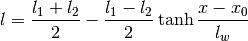Parameters: X : Array, (M,) or scalar float M locations to evaluate length scale at. l1 : positive float Small-X saturation value of the length scale. l2 : positive float Large-X saturation value of the length scale. lw : positive float Length scale of the transition between the two length scales. x0 : float Location of the center of the transition between the two length scales. l : Array, (M,) or scalar float The value of the length scale at the specified point.
gptools.kernel.gibbs.gauss_warp_arb(X, l1, l2, lw, x0)[source]

Warps the X coordinate with a Gaussian-shaped divot.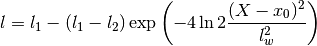Parameters: X : Array, (M,) or scalar float M locations to evaluate length scale at. l1 : positive float Global value of the length scale. l2 : positive float Pedestal value of the length scale. lw : positive float Width of the dip. x0 : float Location of the center of the dip in length scale. l : Array, (M,) or scalar float The value of the length scale at the specified point.
class gptools.kernel.gibbs.GibbsFunction1dArb(warp_function)[source]

Bases: object

Wrapper class for the Gibbs covariance function, permits the use of arbitrary warping.

The covariance function is given by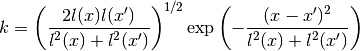Parameters: warp_function : callable The function that warps the length scale as a function of X. Must have the fingerprint (Xi, l1, l2, lw, x0).
__call__(Xi, Xj, sigmaf, l1, l2, lw, x0)[source]

Evaluate the covariance function between points Xi and Xj.

Parameters: Xi, Xj : Array, mpf or scalar float Points to evaluate covariance between. If they are Array, scipy functions are used, otherwise mpmath functions are used. sigmaf : scalar float Prefactor on covariance. l1, l2, lw, x0 : scalar floats Parameters of length scale warping function, passed to warp_function. k : Array or mpf Covariance between the given points.
class gptools.kernel.gibbs.GibbsKernel1dTanhArb(**kwargs)[source]

Gibbs warped squared exponential covariance function in 1d.

Computes derivatives using mpmath.diff() and is hence in general much slower than a hard-coded implementation of a given kernel.

The covariance function is given byWarps the length scale using a hyperbolic tangent:The order of the hyperparameters is:

 0 sigmaf Amplitude of the covariance function 1 l1 Small-X saturation value of the length scale. 2 l2 Large-X saturation value of the length scale. 3 lw Length scale of the transition between the two length scales. 4 x0 Location of the center of the transition between the two length scales.
Parameters: **kwargs All parameters are passed to Kernel.
class gptools.kernel.gibbs.GibbsKernel1dGaussArb(**kwargs)[source]

Gibbs warped squared exponential covariance function in 1d.

Computes derivatives using mpmath.diff() and is hence in general much slower than a hard-coded implementation of a given kernel.

The covariance function is given byWarps the length scale using a gaussian:The order of the hyperparameters is:

 0 sigmaf Amplitude of the covariance function 1 l1 Global value of the length scale. 2 l2 Pedestal value of the length scale. 3 lw Width of the dip. 4 x0 Location of the center of the dip in length scale.
Parameters: **kwargs All parameters are passed to Kernel.
class gptools.kernel.gibbs.GibbsKernel1d(l_func, num_params=None, **kwargs)[source]

Univariate Gibbs kernel with arbitrary length scale warping for low derivatives.

The covariance function is given byThe derivatives are hard-coded using expressions obtained from Mathematica.

Parameters: l_func : callable Function that dictates the length scale warping and its derivative. Must have fingerprint (x, n, p1, p2, ...) where p1 is element one of the kernel’s parameters (i.e., element zero is skipped). num_params : int, optional The number of parameters of the length scale function. If not passed, introspection will be used to determine this. This will fail if you have used the *args syntax in your function definition. This count should include sigma_f, even though it is not passed to the length scale function. **kwargs All remaining arguments are passed to Kernel.
__call__(Xi, Xj, ni, nj, hyper_deriv=None, symmetric=False)[source]

Evaluate the covariance between points Xi and Xj with derivative order ni, nj.

Parameters: Xi : Matrix or other Array-like, (M, D) M inputs with dimension D. Xj : Matrix or other Array-like, (M, D) M inputs with dimension D. ni : Matrix or other Array-like, (M, D) M derivative orders for set i. nj : Matrix or other Array-like, (M, D) M derivative orders for set j. hyper_deriv : Non-negative int or None, optional The index of the hyperparameter to compute the first derivative with respect to. If None, no derivatives are taken. Hyperparameter derivatives are not supported at this point. Default is None. symmetric : bool, optional Whether or not the input Xi, Xj are from a symmetric matrix. Default is False. Kij : Array, (M,) Covariances for each of the M Xi, Xj pairs. NotImplementedError If the hyper_deriv keyword is not None.
gptools.kernel.gibbs.tanh_warp(x, n, l1, l2, lw, x0)[source]

Implements a tanh warping function and its derivative.Parameters: x : float or array of float Locations to evaluate the function at. n : int Derivative order to take. Used for ALL of the points. l1 : positive float Left saturation value. l2 : positive float Right saturation value. lw : positive float Transition width. x0 : float Transition location. l : float or array Warped length scale at the given locations. NotImplementedError If n > 1.
class gptools.kernel.gibbs.GibbsKernel1dTanh(**kwargs)[source]

Gibbs warped squared exponential covariance function in 1d.

Uses hard-coded implementation up to first derivatives.

The covariance function is given byWarps the length scale using a hyperbolic tangent:The order of the hyperparameters is:

 0 sigmaf Amplitude of the covariance function 1 l1 Small-X saturation value of the length scale. 2 l2 Large-X saturation value of the length scale. 3 lw Length scale of the transition between the two length scales. 4 x0 Location of the center of the transition between the two length scales.
Parameters: **kwargs All parameters are passed to Kernel.
gptools.kernel.gibbs.double_tanh_warp(x, n, lcore, lmid, ledge, la, lb, xa, xb)[source]

Implements a sum-of-tanh warping function and its derivative.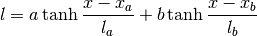Parameters: x : float or array of float Locations to evaluate the function at. n : int Derivative order to take. Used for ALL of the points. lcore : float Core length scale. lmid : float Intermediate length scale. ledge : float Edge length scale. la : positive float Transition of first tanh. lb : positive float Transition of second tanh. xa : float Transition of first tanh. xb : float Transition of second tanh. l : float or array Warped length scale at the given locations. NotImplementedError If n > 1.
class gptools.kernel.gibbs.GibbsKernel1dDoubleTanh(**kwargs)[source]

Gibbs warped squared exponential covariance function in 1d.

Uses hard-coded implementation up to first derivatives.

The covariance function is given byWarps the length scale using two hyperbolic tangents:The order of the hyperparameters is:

 0 sigmaf Amplitude of the covariance function 1 lcore Core length scale 2 lmid Intermediate length scale 3 ledge Edge length scale 4 la Width of first tanh 5 lb Width of second tanh 6 xa Center of first tanh 7 xb Center of second tanh
Parameters: **kwargs All parameters are passed to Kernel.
gptools.kernel.gibbs.cubic_bucket_warp(x, n, l1, l2, l3, x0, w1, w2, w3)[source]

Warps the length scale with a piecewise cubic “bucket” shape.

Parameters: x : float or array-like of float Locations to evaluate length scale at. n : non-negative int Derivative order to evaluate. Only first derivatives are supported. l1 : positive float Length scale to the left of the bucket. l2 : positive float Length scale in the bucket. l3 : positive float Length scale to the right of the bucket. x0 : float Location of the center of the bucket. w1 : positive float Width of the left side cubic section. w2 : positive float Width of the bucket. w3 : positive float Width of the right side cubic section.
class gptools.kernel.gibbs.GibbsKernel1dCubicBucket(**kwargs)[source]

Gibbs warped squared exponential covariance function in 1d.

Uses hard-coded implementation up to first derivatives.

The covariance function is given byWarps the length scale using a “bucket” function with cubic joins.

The order of the hyperparameters is:

 0 sigmaf Amplitude of the covariance function 1 l1 Length scale to the left of the bucket. 2 l2 Length scale in the bucket. 3 l3 Length scale to the right of the bucket. 4 x0 Location of the center of the bucket. 5 w1 Width of the left side cubic section. 6 w2 Width of the bucket. 7 w3 Width of the right side cubic section.
Parameters: **kwargs All parameters are passed to Kernel.
gptools.kernel.gibbs.quintic_bucket_warp(x, n, l1, l2, l3, x0, w1, w2, w3)[source]

Warps the length scale with a piecewise quintic “bucket” shape.

Parameters: x : float or array-like of float Locations to evaluate length scale at. n : non-negative int Derivative order to evaluate. Only first derivatives are supported. l1 : positive float Length scale to the left of the bucket. l2 : positive float Length scale in the bucket. l3 : positive float Length scale to the right of the bucket. x0 : float Location of the center of the bucket. w1 : positive float Width of the left side quintic section. w2 : positive float Width of the bucket. w3 : positive float Width of the right side quintic section.
class gptools.kernel.gibbs.GibbsKernel1dQuinticBucket(**kwargs)[source]

Gibbs warped squared exponential covariance function in 1d.

Uses hard-coded implementation up to first derivatives.

The covariance function is given byWarps the length scale using a “bucket” function with quintic joins.

The order of the hyperparameters is:

 0 sigmaf Amplitude of the covariance function 1 l1 Length scale to the left of the bucket. 2 l2 Length scale in the bucket. 3 l3 Length scale to the right of the bucket. 4 x0 Location of the center of the bucket. 5 w1 Width of the left side quintic section. 6 w2 Width of the bucket. 7 w3 Width of the right side quintic section.
Parameters: **kwargs All parameters are passed to Kernel.
gptools.kernel.gibbs.exp_gauss_warp(X, n, l0, *msb)[source]

Length scale function which is an exponential of a sum of Gaussians.

The centers and widths of the Gaussians are free parameters.

The length scale function is given by

System Message: WARNING/2 (l = l_0 \exp\left ( \sum_{i=1}^{N}eta_i\exp\left ( -)

latex exited with error [stdout] This is pdfTeX, Version 3.1415926-2.5-1.40.14 (TeX Live 2013/Debian) restricted \write18 enabled. entering extended mode (./math.tex LaTeX2e <2011/06/27> Babel <3.9h> and hyphenation patterns for 78 languages loaded. (/usr/share/texlive/texmf-dist/tex/latex/base/article.cls Document Class: article 2007/10/19 v1.4h Standard LaTeX document class (/usr/share/texlive/texmf-dist/tex/latex/base/size12.clo)) (/usr/share/texlive/texmf-dist/tex/latex/base/inputenc.sty (/usr/share/texlive/texmf-dist/tex/latex/ucs/utf8x.def)) (/usr/share/texlive/texmf-dist/tex/latex/ucs/ucs.sty (/usr/share/texlive/texmf-dist/tex/latex/ucs/data/uni-global.def)) (/usr/share/texlive/texmf-dist/tex/latex/amsmath/amsmath.sty For additional information on amsmath, use the ?’ option. (/usr/share/texlive/texmf-dist/tex/latex/amsmath/amstext.sty (/usr/share/texlive/texmf-dist/tex/latex/amsmath/amsgen.sty)) (/usr/share/texlive/texmf-dist/tex/latex/amsmath/amsbsy.sty) (/usr/share/texlive/texmf-dist/tex/latex/amsmath/amsopn.sty)) (/usr/share/texlive/texmf-dist/tex/latex/amscls/amsthm.sty) (/usr/share/texlive/texmf-dist/tex/latex/amsfonts/amssymb.sty (/usr/share/texlive/texmf-dist/tex/latex/amsfonts/amsfonts.sty)) (/usr/share/texlive/texmf-dist/tex/latex/tools/bm.sty) (./math.aux) (/usr/share/texlive/texmf-dist/tex/latex/ucs/ucsencs.def) (/usr/share/texlive/texmf-dist/tex/latex/amsfonts/umsa.fd) (/usr/share/texlive/texmf-dist/tex/latex/amsfonts/umsb.fd) ! Package inputenc Error: Keyboard character used is undefined (inputenc) in inputencoding utf8x’. See the inputenc package documentation for explanation. Type H <return> for immediate help. ... l.14 \end{gather} ! Extra }, or forgotten \right. <template> } $\endtemplate l.14 \end{gather} ! Missing \right. inserted. <inserted text> \right . l.14 \end{gather} ! Missing \right. inserted. <inserted text> \right . l.14 \end{gather} ! Missing } inserted. <inserted text> } l.14 \end{gather} ! Package inputenc Error: Keyboard character used is undefined (inputenc) in inputencoding utf8x’. See the inputenc package documentation for explanation. Type H <return> for immediate help. ... l.14 \end{gather} ! Extra }, or forgotten \right. <template> }$\endtemplate l.14 \end{gather} ! Missing \right. inserted. <inserted text> \right . l.14 \end{gather} ! Missing \right. inserted. <inserted text> \right . l.14 \end{gather} ! Missing } inserted. <inserted text> } l.14 \end{gather}  (./math.aux) ) (see the transcript file for additional information) Output written on math.dvi (1 page, 572 bytes). Transcript written on math.log.

rac{(x-mu_i)^2}{2sigma_i^2} ight ) ight )

The number of parameters is equal to the three times the number of Gaussians plus 1 (for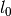). This function is inspired by what Gibbs used in his PhD thesis.
Parameters: X : 1d or 2d array of float The points to evaluate the function at. If 2d, it should only have one column (but this is not checked to save time). n : int The derivative order to compute. Used for all X. l0 : float The covariance length scale at the edges of the domain. *msb : floats Means, standard deviations and weights for each Gaussian, in that order.
class gptools.kernel.gibbs.GibbsKernel1dExpGauss(n_gaussians, **kwargs)[source]

Gibbs warped squared exponential covariance function in 1d.

Uses hard-coded implementation up to first derivatives.

The covariance function is given byWarps the length scale using an exponential of Gaussian basis functions.

The order of the hyperparameters is:

 0 sigmaf Amplitude of the covariance function. 1 l0 Length far away from the Gaussians. 2 mu1 Mean of first Gaussian. 3 mu2 And so on for all Gaussians... 4 sigma1 Width of first Gaussian. 5 sigma2 And so on for all Gaussians... 6 beta1 Amplitude of first Gaussian. 7 beta2 And so on for all Gaussians...
Parameters: n_gaussians : int The number of Gaussian basis functions to use. **kwargs All keywords are passed to Kernel.
class gptools.kernel.gibbs.BSplineWarp(k=3)[source]

Bases: object

Length scale function which is a B-spline.

The degree is fixed at creation, the knot locations and coefficients are free parameters.

Parameters: k : int, optional The polynomial degree to use. Default is 3 (cubic).
__call__(X, n, *tC)[source]

Evaluate the length scale function with the given knots and coefficients.

If X is 2d, uses the first column.

Parameters: X : array of float, (N,) The points to evaluate the length scale function at. n : int The derivative order to compute. *tC : 2M + k - 1 floats The M knots followed by the M + k - 1 coefficients to use.
class gptools.kernel.gibbs.GibbsKernel1dBSpline(nt, k=3, **kwargs)[source]

Gibbs warped squared exponential covariance function in 1d.

Uses hard-coded implementation up to first derivatives.

The covariance function is given byWarps the length scale using a B-spline with free knots but fixed order.

You should always put fixed boundary knots at or beyond the edge of your domain, otherwise the length scale will go to zero. You should always use hyperpriors which keep the coefficients positive, otherwise the length scale can go to zero/be negative.

The order of the hyperparameters is:

 0 sigmaf Amplitude of the covariance function. 1 t1 First knot locations. 2 t2 And so on for all nt knots... 3 C1 First coefficient. 4 C2 And so on for all nt + k - 1 coefficients...
Parameters: nt : int Number of knots to use. Should be at least two (at the edges of the domain of interest). k : int, optional The polynomial degree to use. Default is 3 (cubic). **kwargs All keywords are passed to Kernel.
class gptools.kernel.gibbs.GPWarp(npts, k=None)[source]

Bases: object

Length scale function which is a Gaussian process.

Parameters: npts : int The number of points the GP’s value will be specified on. k : Kernel instance, optional The covariance kernel to use for the GP. The default is to use a SquaredExponentialKernel with fixed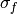(since it does not matter here) and broad bounds on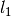.
__call__(X, n, *hpXy)[source]

Evaluate the length scale.

Parameters: X : array of float The points to evaluate the length scale at. n : int The order of derivative to compute. *hpXy : floats The free hyperparameters of the GP, then the points to set the value at, then the values to use.
class gptools.kernel.gibbs.GibbsKernel1dGP(npts, k=None, **kwargs)[source]

Gibbs warped squared exponential covariance function in 1d.

Uses hard-coded implementation up to first derivatives.

The covariance function is given byWarps the length scale using a Gaussian process which interpolates the values specified at a set number of points. Both the values and the locations of the points can be treated as free parameters.

You should try to pick a hyperprior which keeps the outer points close to the edge of the domain, as otherwise the Gaussian process will try to go to zero there. You should put a hyperprior on the values at the points which keeps them positive, otherwise unphysical length scales will result.

The order of the hyperparameters is:

 0 sigmaf Amplitude of the covariance function. 1 hp1 First hyperparameter of the covariance function. 2 hp2 And so on for all free hyperparameters... 3 x1 Location of the first point to set the value at. 4 x2 And so on for all points the value is set at... 5 y1 Length scale at the first point. 6 y2 And so on for all points the value is set at...
Parameters: npts : int Number of points to use. k : Kernel instance, optional The covariance kernel to use for the GP. The default is to use a SquaredExponentialKernel with fixed(since it does not matter here) and broad bounds on. **kwargs All keywords are passed to Kernel.

## gptools.kernel.matern module¶

Provides the MaternKernel class which implements the anisotropic Matern kernel.

gptools.kernel.matern.matern_function(Xi, Xj, *args)[source]

Matern covariance function of arbitrary dimension, for use with ArbitraryKernel.

The Matern kernel has the following hyperparameters, always referenced in the order listed:

 0 sigma prefactor 1 nu order of kernel 2 l1 length scale for the first dimension 3 l2 ...and so on for all dimensions

The kernel is defined as: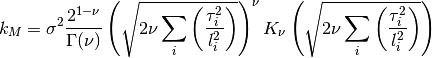Parameters: Xi, Xj : Array, mpf, tuple or scalar float Points to evaluate the covariance between. If they are Array, scipy functions are used, otherwise mpmath functions are used. *args Remaining arguments are the 2+num_dim hyperparameters as defined above.
class gptools.kernel.matern.MaternKernelArb(**kwargs)[source]

Matern covariance kernel. Supports arbitrary derivatives. Treats order as a hyperparameter.

This version of the Matern kernel is painfully slow, but uses mpmath to ensure the derivatives are computed properly, since there may be issues with the regular MaternKernel.

The Matern kernel has the following hyperparameters, always referenced in the order listed:

 0 sigma prefactor 1 nu order of kernel 2 l1 length scale for the first dimension 3 l2 ...and so on for all dimensions

The kernel is defined as:Parameters: **kwargs All keyword parameters are passed to ArbitraryKernel.
nu

Returns the value of the order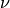.

class gptools.kernel.matern.MaternKernel1d(**kwargs)[source]

Matern covariance kernel. Only for univariate data. Only supports up to first derivatives. Treats order as a hyperparameter.

The Matern kernel has the following hyperparameters, always referenced in the order listed:

 0 sigma prefactor 1 nu order of kernel 2 l1 length scale

The kernel is defined as: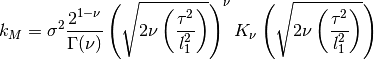where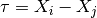.

Note that the expressions for the derivatives break down for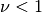.

Parameters: **kwargs All keyword parameters are passed to Kernel.
__call__(Xi, Xj, ni, nj, hyper_deriv=None, symmetric=False)[source]

Evaluate the covariance between points Xi and Xj with derivative order ni, nj.

Parameters: Xi : Matrix or other Array-like, (M, D) M inputs with dimension D. Xj : Matrix or other Array-like, (M, D) M inputs with dimension D. ni : Matrix or other Array-like, (M, D) M derivative orders for set i. nj : Matrix or other Array-like, (M, D) M derivative orders for set j. hyper_deriv : Non-negative int or None, optional The index of the hyperparameter to compute the first derivative with respect to. If None, no derivatives are taken. Hyperparameter derivatives are not supported at this point. Default is None. symmetric : bool, optional Whether or not the input Xi, Xj are from a symmetric matrix. Default is False. Kij : Array, (M,) Covariances for each of the M Xi, Xj pairs. NotImplementedError If the hyper_deriv keyword is not None.
class gptools.kernel.matern.MaternKernel(num_dim=1, **kwargs)[source]

Matern covariance kernel. Supports arbitrary derivatives. Treats order as a hyperparameter.

The Matern kernel has the following hyperparameters, always referenced in the order listed:

 0 sigma prefactor 1 nu order of kernel 2 l1 length scale for the first dimension 3 l2 ...and so on for all dimensions

The kernel is defined as:Parameters: num_dim : int Number of dimensions of the input data. Must be consistent with the X and Xstar values passed to the GaussianProcess you wish to use the covariance kernel with. **kwargs All keyword parameters are passed to ChainRuleKernel. ValueError If num_dim is not a positive integer or the lengths of the input vectors are inconsistent. GPArgumentError If fixed_params is passed but initial_params is not.
nu

Returns the value of the order.

class gptools.kernel.matern.Matern52Kernel(num_dim=1, **kwargs)[source]

Matern 5/2 covariance kernel. Supports only 0th and 1st derivatives and is fixed at nu=5/2. Because of these limitations, it is quite a bit faster than the more general Matern kernels.

The Matern52 kernel has the following hyperparameters, always referenced in the order listed:

 0 sigma prefactor 1 l1 length scale for the first dimension 2 l2 ...and so on for all dimensions

The kernel is defined as: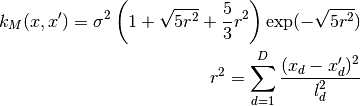Parameters: num_dim : int Number of dimensions of the input data. Must be consistent with the X and Xstar values passed to the GaussianProcess you wish to use the covariance kernel with. **kwargs All keyword parameters are passed to Kernel. ValueError If num_dim is not a positive integer or the lengths of the input vectors are inconsistent. GPArgumentError If fixed_params is passed but initial_params is not.
__call__(Xi, Xj, ni, nj, hyper_deriv=None, symmetric=False)[source]

Evaluate the covariance between points Xi and Xj with derivative order ni, nj.

Parameters: Xi : Matrix or other Array-like, (M, D) M inputs with dimension D. Xj : Matrix or other Array-like, (M, D) M inputs with dimension D. ni : Matrix or other Array-like, (M, D) M derivative orders for set i. nj : Matrix or other Array-like, (M, D) M derivative orders for set j. hyper_deriv : Non-negative int or None, optional The index of the hyperparameter to compute the first derivative with respect to. If None, no derivatives are taken. Hyperparameter derivatives are not supported at this point. Default is None. symmetric : bool Whether or not the input Xi, Xj are from a symmetric matrix. Default is False. Kij : Array, (M,) Covariances for each of the M Xi, Xj pairs. NotImplementedError If the hyper_deriv keyword is not None.

## gptools.kernel.noise module¶

Provides classes for implementing uncorrelated noise.

class gptools.kernel.noise.DiagonalNoiseKernel(num_dim=1, initial_noise=None, fixed_noise=False, noise_bound=None, n=0, hyperprior=None)[source]

Kernel that has constant, independent noise (i.e., a diagonal kernel).

Parameters: num_dim : positive int Number of dimensions of the input data. initial_noise : float, optional Initial value for the noise standard deviation. Default value is None (noise gets set to 1). fixed_noise : bool, optional Whether or not the noise is taken to be fixed when optimizing the log likelihood. Default is False (noise is not fixed). noise_bound : 2-tuple, optional The bounds for the noise when optimizing the log likelihood with scipy.optimize.minimize(). Must be of the form (lower, upper). Set a given entry to None to put no bound on that side. Default is None, which gets set to (0, None). n : non-negative int or tuple of non-negative ints with length equal to num_dim, optional Indicates which derivative this noise is with respect to. Default is 0 (noise applies to value). hyperprior : callable, optional Function that returns the prior log-density for a possible value of noise when called. Must also have an attribute called bounds that is the bounds on the noise and a method called random_draw() that yields a random draw. Default behavior is to assign a uniform prior.
__call__(Xi, Xj, ni, nj, hyper_deriv=None, symmetric=False)[source]

Evaluate the covariance between points Xi and Xj with derivative order ni, nj.

Parameters: Xi : Matrix or other Array-like, (M, D) M inputs with dimension D. Xj : Matrix or other Array-like, (M, D) M inputs with dimension D. ni : Matrix or other Array-like, (M, D) M derivative orders for set i. nj : Matrix or other Array-like, (M, D) M derivative orders for set j. hyper_deriv : Non-negative int or None, optional The index of the hyperparameter to compute the first derivative with respect to. Since this kernel only has one hyperparameter, 0 is the only valid value. If None, no derivatives are taken. Default is None (no hyperparameter derivatives). symmetric : bool, optional Whether or not the input Xi, Xj are from a symmetric matrix. Default is False. Kij : Array, (M,) Covariances for each of the M Xi, Xj pairs.
class gptools.kernel.noise.ZeroKernel(num_dim=1)[source]

Kernel that always evaluates to zero, used as the default noise kernel.

Parameters: num_dim : positive int The number of dimensions of the inputs.
__call__(Xi, Xj, ni, nj, hyper_deriv=None, symmetric=False)[source]

Return zeros the same length as the input Xi.

Ignores all other arguments.

Parameters: Xi : Matrix or other Array-like, (M, D) M inputs with dimension D. Xj : Matrix or other Array-like, (M, D) M inputs with dimension D. ni : Matrix or other Array-like, (M, D) M derivative orders for set i. nj : Matrix or other Array-like, (M, D) M derivative orders for set j. hyper_deriv : Non-negative int or None, optional The index of the hyperparameter to compute the first derivative with respect to. Since this kernel only has one hyperparameter, 0 is the only valid value. If None, no derivatives are taken. Default is None (no hyperparameter derivatives). symmetric : bool, optional Whether or not the input Xi, Xj are from a symmetric matrix. Default is False. Kij : Array, (M,) Covariances for each of the M Xi, Xj pairs.

Provides the RationalQuadraticKernel class which implements the anisotropic rational quadratic (RQ) kernel.

class gptools.kernel.rational_quadratic.RationalQuadraticKernel(num_dim=1, **kwargs)[source]

Rational quadratic (RQ) covariance kernel. Supports arbitrary derivatives.

The RQ kernel has the following hyperparameters, always referenced in the order listed:

 0 sigma prefactor. 1 alpha order of kernel. 2 l1 length scale for the first dimension. 3 l2 ...and so on for all dimensions.

The kernel is defined as: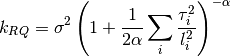Parameters: num_dim : int Number of dimensions of the input data. Must be consistent with the X and Xstar values passed to the GaussianProcess you wish to use the covariance kernel with. **kwargs All keyword parameters are passed to ChainRuleKernel. ValueError If num_dim is not a positive integer or the lengths of the input vectors are inconsistent. GPArgumentError If fixed_params is passed but initial_params is not.

## gptools.kernel.squared_exponential module¶

Provides the SquaredExponentialKernel class that implements the anisotropic SE kernel.

class gptools.kernel.squared_exponential.SquaredExponentialKernel(num_dim=1, **kwargs)[source]

Squared exponential covariance kernel. Supports arbitrary derivatives.

Supports derivatives with respect to the hyperparameters.

The squared exponential has the following hyperparameters, always referenced in the order listed:

 0 sigma prefactor on the SE 1 l1 length scale for the first dimension 2 l2 ...and so on for all dimensions

The kernel is defined as: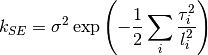Parameters: num_dim : int Number of dimensions of the input data. Must be consistent with the X and Xstar values passed to the GaussianProcess you wish to use the covariance kernel with. **kwargs All keyword parameters are passed to Kernel. ValueError If num_dim is not a positive integer or the lengths of the input vectors are inconsistent. GPArgumentError If fixed_params is passed but initial_params is not.
__call__(Xi, Xj, ni, nj, hyper_deriv=None, symmetric=False)[source]

Evaluate the covariance between points Xi and Xj with derivative order ni, nj.

Parameters: Xi : Matrix or other Array-like, (M, D) M inputs with dimension D. Xj : Matrix or other Array-like, (M, D) M inputs with dimension D. ni : Matrix or other Array-like, (M, D) M derivative orders for set i. nj : Matrix or other Array-like, (M, D) M derivative orders for set j. hyper_deriv : Non-negative int or None, optional The index of the hyperparameter to compute the first derivative with respect to. If None, no derivatives are taken. Default is None (no hyperparameter derivatives). Hyperparameter derivatives are not support for n > 0 at this time. symmetric : bool, optional Whether or not the input Xi, Xj are from a symmetric matrix. Default is False. Kij : Array, (M,) Covariances for each of the M Xi, Xj pairs.

## gptools.kernel.warping module¶

Classes and functions to warp inputs to kernels to enable fitting of nonstationary data. Note that this accomplishes a similar goal as the Gibbs kernel (which is a nonstationary version of the squared exponential kernel), but with the difference that the warpings in this module can be applied to any existing kernel. Furthermore, whereas the Gibbs kernel implements nonstationarity by changing the length scale of the covariance function, the warpings in the module act by transforming the input values directly.

The module contains two core classes that work together. WarpingFunction gives you a way to wrap a given function in a way that allows you to optimize/integrate over the hyperparameters that describe the warping. WarpedKernel is an extension of the Kernel base class and is how you apply a WarpingFunction to whatever kernel you want to warp.

Two useful warpings have been implemented at this point: BetaWarpedKernel warps the inputs using the CDF of the beta distribution (i.e., the regularized incomplete beta function). This requires that the starting inputs be constrained to the unit hypercube [0, 1]. In order to get arbitrary data to this form, LinearWarpedKernel allows you to apply a linear transformation based on the known bounds of your data.

So, for example, to make a beta-warped squared exponential kernel, you simply type:

k_SE = gptools.SquaredExponentialKernel()
k_SE_beta = gptools.BetaWarpedKernel(k_SE)


Furthermore, if your inputs X are not confined to the unit hypercube [0, 1], you should use a linear transformation to map them to it:

k_SE_beta_unit = gptools.LinearWarpedKernel(k_SE_beta, X.min(axis=0), X.max(axis=0))

class gptools.kernel.warping.WarpingFunction(fun, num_dim=1, num_params=None, initial_params=None, fixed_params=None, param_bounds=None, param_names=None, enforce_bounds=False, hyperprior=None)[source]

Bases: object

Wrapper to interface a function with WarpedKernel.

This lets you define a simple function fun(X, d, n, p1, p2, ...) that operates on one dimension of X at a time and has several hyperparameters.

Parameters: fun : callable Must have fingerprint fun(X, d, n, p1, p2, ...) where X is an array of length M, d is the index of the dimension X is from, n is a non-negative integer representing the order of derivative to take and p1, p2, ... are the parameters of the warping. Note that this form assumes that the warping is applied independently to each dimension. num_dim : positive int, optional Number of dimensions of the input data. Must be consistent with the X and Xstar values passed to the GaussianProcess you wish to use the warping function with. Default is 1. num_params : Non-negative int, optional Number of parameters in the model. Default is to determine the number of parameters by inspection of fun or the other arguments provided. initial_params : Array, (num_params,), optional Initial values to set for the hyperparameters. Default is None, in which case 1 is used for the initial values. fixed_params : Array of bool, (num_params,), optional Sets which hyperparameters are considered fixed when optimizing the log likelihood. A True entry corresponds to that element being fixed (where the element ordering is as defined in the class). Default value is None (no hyperparameters are fixed). param_bounds : list of 2-tuples (num_params,), optional List of bounds for each of the hyperparameters. Each 2-tuple is of the form (lower, upper). If there is no bound in a given direction, it works best to set it to something big like 1e16. Default is (0.0, 1e16) for each hyperparameter. Note that this is overridden by the hyperprior keyword, if present. param_names : list of str (num_params,), optional List of labels for the hyperparameters. Default is all empty strings. enforce_bounds : bool, optional If True, an attempt to set a hyperparameter outside of its bounds will result in the hyperparameter being set right at its bound. If False, bounds are not enforced inside the kernel. Default is False (do not enforce bounds). hyperprior : JointPrior instance or list, optional Joint prior distribution for all hyperparameters. Can either be given as a JointPrior instance or a list of num_params callables or rv_frozen instances from scipy.stats, in which case a IndependentJointPrior is constructed with these as the independent priors on each hyperparameter. Default is a uniform PDF on all hyperparameters.
__call__(X, d, n)[source]

Evaluate the warping function.

Parameters: X : Array, (M,) M inputs corresponding to dimension d. d : non-negative int Index of the dimension that X is from. n : non-negative int Derivative order to compute.
param_bounds
set_hyperparams(new_params)[source]

Sets the free hyperparameters to the new parameter values in new_params.

Parameters: new_params : Array or other Array-like, (len(self.params),) New parameter values, ordered as dictated by the docstring for the class.
num_free_params

Returns the number of free parameters.

free_param_idxs

Returns the indices of the free parameters in the main arrays of parameters, etc.

free_params

Returns the values of the free hyperparameters.

Returns: free_params : Array Array of the free parameters, in order.
free_param_bounds

Returns the bounds of the free hyperparameters.

Returns: free_param_bounds : Array Array of the bounds of the free parameters, in order.
free_param_names

Returns the names of the free hyperparameters.

Returns: free_param_names : Array Array of the names of the free parameters, in order.
gptools.kernel.warping.beta_cdf_warp(X, d, n, *args)[source]

Warp inputs that are confined to the unit hypercube using the regularized incomplete beta function.

Applies separately to each dimension, designed for use with WarpingFunction.

Assumes that your inputs X lie entirely within the unit hypercube [0, 1].

Note that you may experience some issues with constraining and computing derivatives at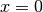when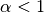and at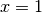when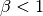. As a workaround, try mapping your data to not touch the boundaries of the unit hypercube.

Parameters: X : array, (M,) M inputs from dimension d. d : non-negative int The index (starting from zero) of the dimension to apply the warping to. n : non-negative int The derivative order to compute. *args : 2N scalars The remaining parameters to describe the warping, given as scalars. These are given as alpha_i, beta_i for each of the D dimensions. Note that these must ALL be provided for each call.

References

 [R1] J. Snoek, K. Swersky, R. Zemel, R. P. Adams, “Input Warping for Bayesian Optimization of Non-stationary Functions” ICML (2014)
gptools.kernel.warping.linear_warp(X, d, n, *args)[source]

Warp inputs with a linear transformation.

Applies the warping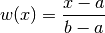to each dimension. If you set a=min(X) and b=max(X) then this is a convenient way to map your inputs to the unit hypercube.

Parameters: X : array, (M,) M inputs from dimension d. d : non-negative int The index (starting from zero) of the dimension to apply the warping to. n : non-negative int The derivative order to compute. *args : 2N scalars The remaining parameters to describe the warping, given as scalars. These are given as a_i, b_i for each of the D dimensions. Note that these must ALL be provided for each call.
class gptools.kernel.warping.ISplineWarp(nt, k=3)[source]

Bases: object

Warps inputs with an I-spline.

Applies the warping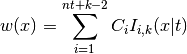to each dimension, where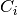are the coefficients and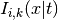are the I-spline basis functions with knot grid.

Parameters: nt : int or array of int (D,) The number of knots. If this is a single int, it is used for all of the dimensions. If it is an array of ints, it is the number of knots for each dimension. k : int, optional The polynomial degree of I-spline to use. The same degree is used for all dimensions. The default is 3 (cubic I-splines).
__call__(X, d, n, *args)[source]

Evaluate the I-spline warping function.

Parameters: X : array, (M,) M inputs from dimension d. d : non-negative int The index (starting from zero) of the dimension to apply the warping to. n : non-negative int The derivative order to compute. *args : scalar flots The remaining parameters to describe the warping, given as scalars. These are given as the knots followed by the coefficients, for each dimension. Note that these must ALL be provided for each call.
class gptools.kernel.warping.WarpedKernel(k, w)[source]

Kernel which has had its inputs warped through a basic, elementwise warping function.

In other words, takes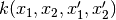and turns it into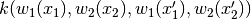.

__call__(Xi, Xj, ni, nj, hyper_deriv=None, symmetric=False)[source]
w_func(X, d, n)[source]

Evaluate the (possibly recursive) warping function and its derivatives.

Parameters: X : array, (M,) The points (from dimension d) to evaluate the warping function at. d : int The dimension to warp. n : int The derivative order to compute. So far only 0 and 1 are supported.
enforce_bounds

Boolean indicating whether or not the kernel will explicitly enforce the bounds defined by the hyperprior.

fixed_params
params
param_names
free_params
free_param_bounds
free_param_names
set_hyperparams(new_params)[source]

Set the (free) hyperparameters.

Parameters: new_params : Array or other Array-like New values of the free parameters. ValueError If the length of new_params is not consistent with self.params.
class gptools.kernel.warping.BetaWarpedKernel(k, **w_kwargs)[source]

Class to warp any existing Kernel with the beta CDF.

Assumes that your inputs X lie entirely within the unit hypercube [0, 1].

Note that you may experience some issues with constraining and computing derivatives atwhenand atwhen. As a workaround, try mapping your data to not touch the boundaries of the unit hypercube.

Parameters: k : Kernel The Kernel to warp. **w_kwargs : optional kwargs All additional kwargs are passed to the constructor of WarpingFunction. If no hyperprior or param_bounds are provided, takes each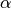,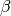to follow the log-normal distribution.

References

 [R2] J. Snoek, K. Swersky, R. Zemel, R. P. Adams, “Input Warping for Bayesian Optimization of Non-stationary Functions” ICML (2014)
class gptools.kernel.warping.LinearWarpedKernel(k, a, b)[source]

Class to warp any existing Kernel with the linear transformation given in linear_warp().

If you set a to be the minimum of your X inputs in each dimension and b to be the maximum then you can use this to map data from an arbitrary domain to the unit hypercube [0, 1], as is required for application of the BetaWarpedKernel, for instance.

Parameters: k : Kernel The Kernel to warp. a : list The a parameter in the linear warping defined in linear_warp(). This list must have length equal to k.num_dim. b : list The b parameter in the linear warping defined in linear_warp(). This list must have length equal to k.num_dim.
class gptools.kernel.warping.ISplineWarpedKernel(k, nt, k_deg=3, **w_kwargs)[source]

Class to warp any existing Kernel with the I-spline transformation given in ISplineWarp().

Parameters: k : Kernel The Kernel to warp. nt : int or array of int The number of knots. If this is a single int, it is used for all of the dimensions. If it is an array of ints, it is the number of knots for each dimension. k_deg : int, optional The polynomial degree of I-spline to use. The same degree is used for all dimensions. The default is 3 (cubic I-splines). **w_kwargs : optional kwargs All additional keyword arguments are passed to the constructor of WarpingFunction.

## Module contents¶

Subpackage containing a variety of covariance kernels and associated helpers.